Courses

# Test: Shear

## 10 Questions MCQ Test GATE Civil Engineering (CE) 2022 Mock Test Series | Test: Shear

Description
This mock test of Test: Shear for Civil Engineering (CE) helps you for every Civil Engineering (CE) entrance exam. This contains 10 Multiple Choice Questions for Civil Engineering (CE) Test: Shear (mcq) to study with solutions a complete question bank. The solved questions answers in this Test: Shear quiz give you a good mix of easy questions and tough questions. Civil Engineering (CE) students definitely take this Test: Shear exercise for a better result in the exam. You can find other Test: Shear extra questions, long questions & short questions for Civil Engineering (CE) on EduRev as well by searching above.
QUESTION: 1

### For a reinforced concrete section, the shape of shear stress diagram is

Solution: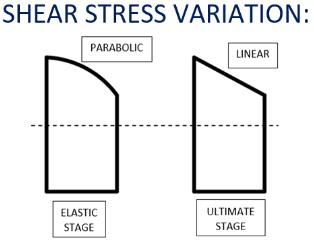QUESTION: 2

### The ratio of permissible shear stress in limit state method of design and working stress method of design is

Solution:

Permissible shear stress.
In limit state method of design,
τc = 0.25√fck
in working state method of design,
τc = 0.16√fck
∴ Ratio = 25/16

QUESTION: 3

### The contribution of bent up bars towards shear resistance shall not be more than

Solution:

Total shear strength = shear resistance of effective concrete area as a function of longitudinal bars + shear resistance of vertical shear stirrups + shear resistance of-inclined shear stirrups.
Tests have shown that inclined bars alone do not provide a satisfactory solution and their contribution is limited to 50% of net shear strength after deducting the contribution of concrete. The remaining shear resistance is provided by vertical stirrups.

QUESTION: 4

If the nominal shear stress (ζv) at a section does not exceed the permissible shear stress (τc)

Solution:

If the calculated shear stress ( ζ​v ) is
(i) less than allowable shear stress (ζ​c) but more than 0.5ζ​c, the minimum shear reinforcement in the form of stirrups shall be provided such that,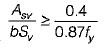(ii) more than ζ​c, shear reinforcement shall be provided in the form of vertical stirrups or bent up bars with stirrups or inclined stirrups to resist
Vus = Vu - τcbd. Here  τv < τc,max
(iii) If  τv >  τc,max redesign the section.

QUESTION: 5

A beam of rectangular cross-section (b x d) is subjected to a torque T. What is the maximum torsional stress induced in the beam (b < d and α is a constant)?

Solution:

Torsion constant of a rectangular section of width b and depth d (b < d) may be expressed as,
J = b3d

For T, L and / sections torsion constant,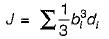where bi and di are the dimensions of each of the component rectangles into which the section may be divided.
Torsional shear stress for rectangular section

τ = T/αb2d

For T, L and / sections torsional shear stress may be calculated for each component rectangle by considering them subjected to torsional moment,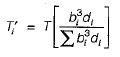QUESTION: 6

The minimum cover in a slab should neither be less than the diameter of bar nor less than

Solution: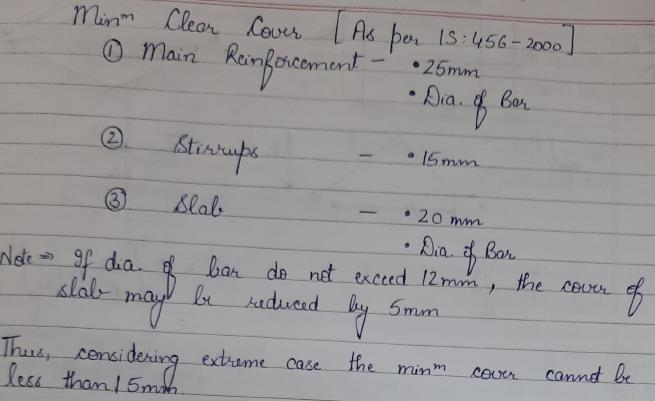QUESTION: 7

The average permissible stress in bond for plain bars in tension is

Solution:
QUESTION: 8

When shear stress exceeds the permissible limit in a slab, then it is reduced by

Solution:

Shear stress in slabs is controlled by increasing the depth of slab and not shear reinforcement while in beams shear stirrups are provided to control shear.

QUESTION: 9

Flexural shear failure in a reinforced concrete beam
1. occurs under large bending moment and less shearforce
2. results in cracks which are normally at 90° with the horizontal
3. occurs when shear span to effective depth ratio is less than 1

Which of these statements are correct?

Solution:
QUESTION: 10

For M 20 grade of concrete, the maximum shear stress shall not exceed

Solution: